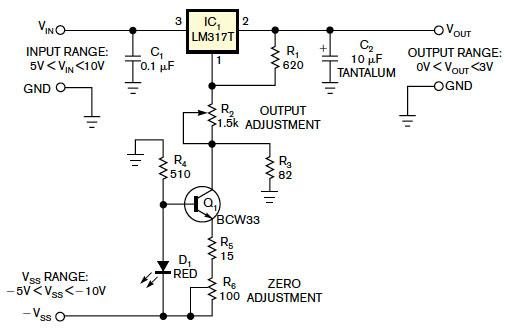# Use an LM317 as 0 to 3V adjustable regulatorFigure 1 This circuit is an inexpensive approach using a simple 0 to 3V adjustable regulator.

READ  They act only ones – right then, when it´s really necessary

Figure 1 shows an inexpensive approach using a simple 0 to 3V adjustable regulator. You implement the necessary potential bias using a simple temperature-stabilized constant-current source (Reference 4). You calculate this current source using the following equation: I=(VF–VEBO)/(R5+R6), where VF is D1’s forward voltage of approximately 2V and VEBO is Q1’s emitter-base voltage of approximately 0.68V. The current is approximately 1.32/(R5+R6). The constant-current source creates a bias voltage of approximately –1.25V on resistor R3. You implement the zero adjustment using resistor R6, which can change the current of the constant-current source. Resistor R5 protects transistor Q1. You can use D1 as a light indicator. You can adjust the output voltage using resistor R2. Calculate the output voltage as follows: VOUT=VREF(1+R2/R1)–VR3, where VREF is the reference voltage of IC1 and VR3 is some compensative voltage of resistor R3. You should establish this voltage to equal the reference voltage for its compensation. In this case, VOUT=VREF(R2/R1). With R2 having a value of 1.2 kΩ,this circuit found use as the equivalent of a typical battery with an output voltage of 1.56V for development projects.

For more detail:Use an LM317 as 0 to 3V adjustable regulator